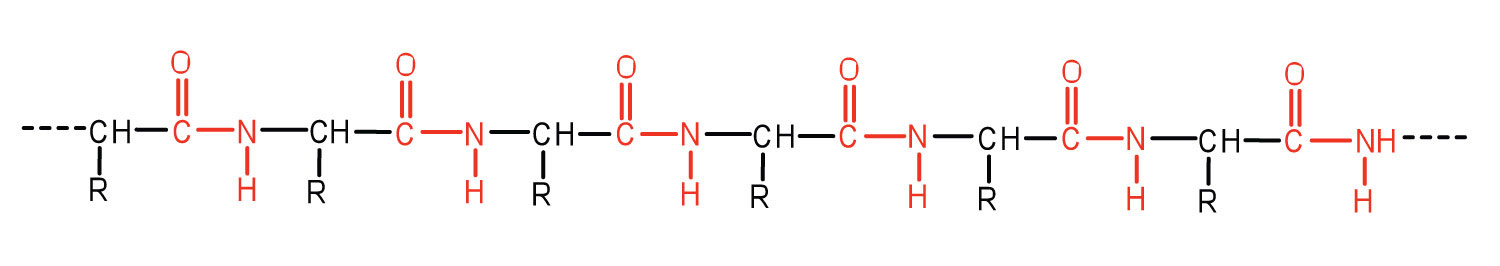# Question #e52b8

Jun 8, 2015

The DDT would be found in the fat, because both DDT and fat are mostly nonpolar molecules.

#### Explanation:

The structure of DDT isDDT appears to have many polar $\text{C-Cl}$ bonds, but you must remember that a $\text{C-Cl}$ bond is only slightly more polar than a $\text{C-H}$ bond (Δ EN = 0.6 vs. 0.4), and the molecule consists mostly of $\text{C-C}$ and $\text{C-H}$ bonds.

So, DDT is essentially a nonpolar molecule.

We know that Like dissolves like. That is, nonpolar solutes dissolve in nonpolar solvents.

Let's look at the structures of the three milk components and decide on their polarities.

The structure of a typical milk carbohydrate isIt has many polar $\text{OH}$ groups. Nonpolar DDT will not dissolve in milk carbohydrates.

Casein is a typical milk protein. Its structure isWe see that it contains many polar $\text{C=O}$ and $\text{N-H}$ groups. Nonpolar DDT will not dissolve in milk proteins.

The structure of a typical milk fat isWe see that, except for a few $\text{C=O}$ and $\text{C-O}$ groups, it consists mostly of nonpolar $\text{C-C}$ and $\text{C-H}$ bonds.

The fat is almost completely nonpolar. DDT will dissolve in the milk fats.